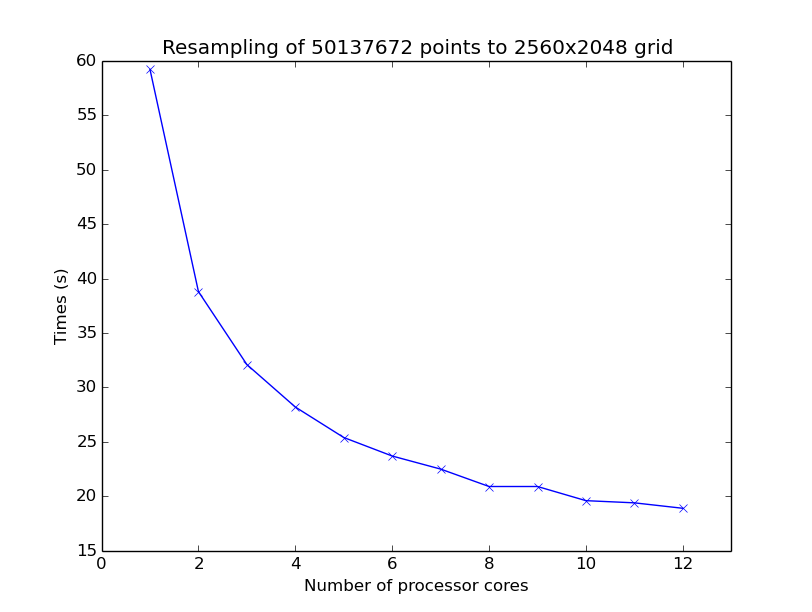# Using multiple processor cores¶

## Multi core processing¶

Bottlenecks of pyresample can be executed in parallel. Parallel computing can be executed if the pyresample function has the nprocs keyword argument. nprocs specifies the number of processes to be used for calculation. If a class takes the constructor argument nprocs this sets nprocs for all methods of this class

Example of resampling in parallel using 4 processes:

```>>> import numpy
>>> from pyresample import kd_tree, geometry
...                                {'a': '6378144.0', 'b': '6356759.0',
...                                 'lat_0': '50.00', 'lat_ts': '50.00',
...                                 'lon_0': '8.00', 'proj': 'stere'},
...                                800, 800,
...                                [-1370912.72, -909968.64,
...                                 1029087.28, 1490031.36])
>>> data = numpy.fromfunction(lambda y, x: y*x, (50, 10))
>>> lons = numpy.fromfunction(lambda y, x: 3 + x, (50, 10))
>>> lats = numpy.fromfunction(lambda y, x: 75 - y, (50, 10))
>>> swath_def = geometry.SwathDefinition(lons=lons, lats=lats)
>>> result = kd_tree.resample_nearest(swath_def, data.ravel(),